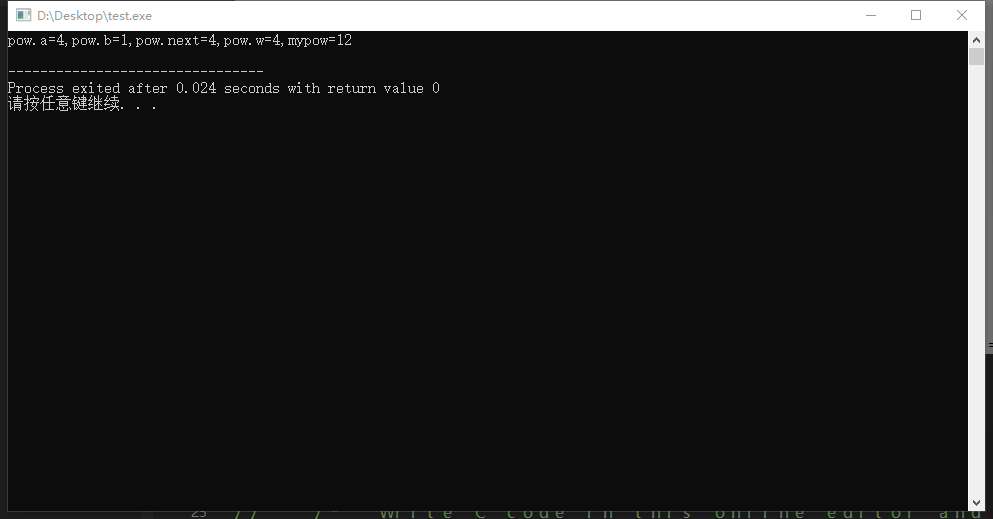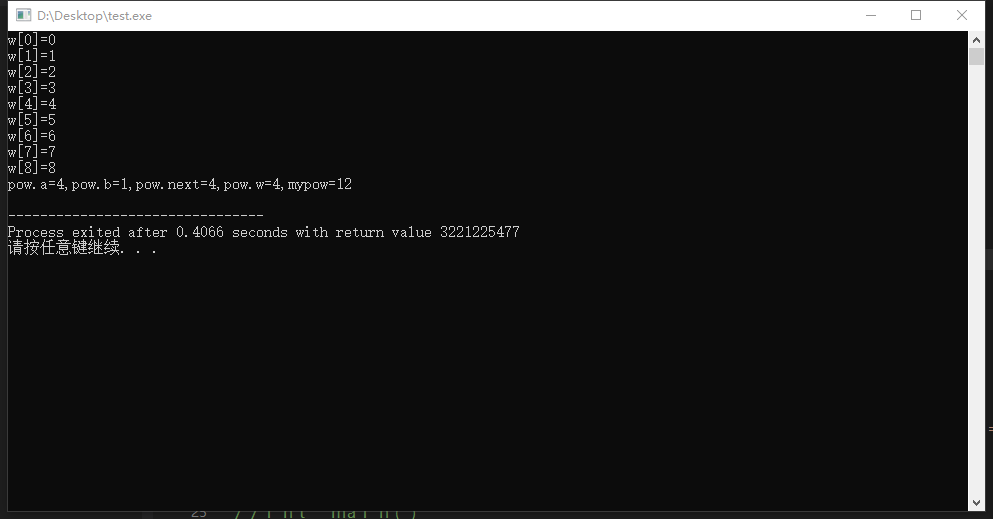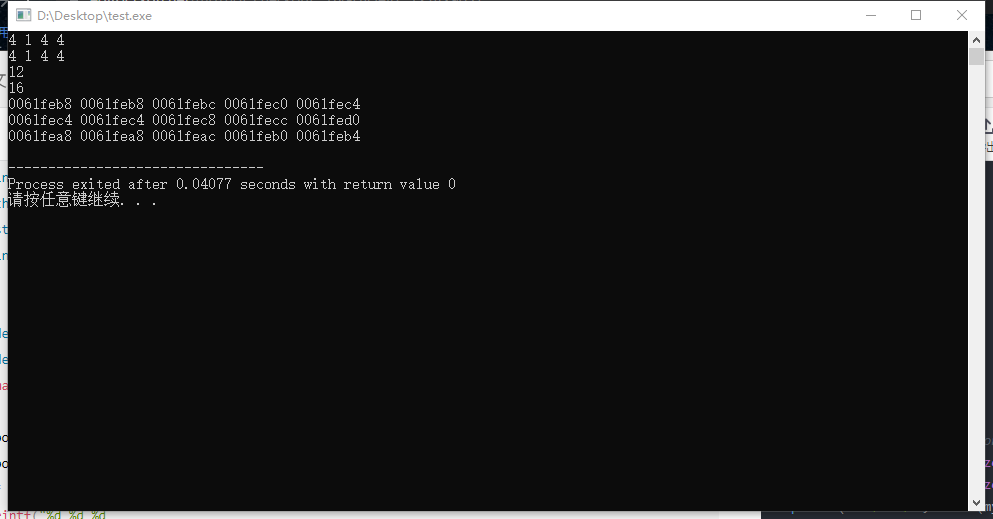# 一、这篇文章说的是什么（有基础的可以不看）

#define MAX 50;
struct apow {
int a ;
char b;
struct pow *next;
int w[MAX];//开辟50个元素的数组
};//直接确定数组大小

struct pows {
int  a;
char b;
struct pows *next;
int *w;
};//动态开辟内存空间

struct pow {
int a ;
char b;
struct pow *next;
int w[];
};//将数组作为结构体的成员进行声明时元素个数可以不定义（只需要写[]）


# 二、使用结构体中的灵活数组的陷阱

#include <stdio.h>
struct pow {
int a ;
char b;
struct pow *next;
int w[];
};
int main(){
struct pow mypow;	printf("pow.a=%d,pow.b=%d,pow.next=%d,pow.w=%d,mypow=%d\n",sizeof(mypow.a),sizeof(mypow.b),sizeof(mypow.next),sizeof(mypow.w),sizeof(mypow));
}#include <stdio.h>
struct pow {
int a ;
char b;
struct pow *next;
int w[];
};
struct pows {
int  a;
char b;
struct pows *next;
int *w;
};

int main(){
struct pow mypow;
for(int i=0;i<9;i++){
mypow.w[i]=i;
printf("w[%d]=%d\n",i,mypow.w[i]);
}
printf("pow.a=%d,pow.b=%d,pow.next=%d,pow.w=%d,mypow=%d\n",sizeof(mypow.a),sizeof(mypow.b),sizeof(mypow.next),sizeof(mypow.w),sizeof(mypow));
}#include <stdio.h>
struct pow {
int a ;
char b;
struct pow *next;
int w[];
};
struct pows {
int  a;
char b;
struct pows *next;
int *w;
};

typedef struct pow pow_t;
typedef struct pows pows_t;
int main()
{
pow_t mypow;
pows_t mypows;
/*  Write C code in this online editor and run it. */
printf("%d %d %d %d\n",sizeof(mypow.a),sizeof(mypow.b),sizeof(mypow.next),sizeof(mypow.w));
printf("%d %d %d %d\n",sizeof(mypows.a),sizeof(mypows.b),sizeof(mypows.next),sizeof(mypows.w));
printf("%d\n%d\n",sizeof(mypow),sizeof(mypows));
printf("%p %p %p %p %p\n",&mypow,&mypow.a,&mypow.b,&mypow.next,&mypow.w);
printf("%p %p %p %p %p\n",&mypow,&mypow.a,&mypow.b,&mypow.next,mypow.w);
printf("%p %p %p %p %p\n",&mypows,&mypows.a,&mypows.b,&mypows.next,&mypows.w);
return 0;
}# 总结

1、sizeof不是函数，是关键字，在程序运行之前就完成了测算然后已经装入了。
2、使用“将数组作为结构体的成员进行声明时元素个数可以不定义（只需要写[]）”时，需要注意内存泄露问题，这就是它最大的陷阱。
3、虽然可以使用，对于c语言是合法的，但是操作对于内存来说是不合法的。

hhh，作者是个小菜鸡，争取把自己犯的错，都记下来以后避免，当然在学习的过程中可能会有很多错误，欢迎大家评论区批评指出。